Flags

# What to expect in your numerical aptitude test including test questions examples

In the numerical aptitude test question, you are given numerical information in a table, graph or number series that describes a business-related situation. Each question has between four and five optional answers. Your task is to decide which is the correct answer based solely on the numerical information presented.

Below are three examples of three types of numerical aptitude test questions. You can click on the image to enlarge.

•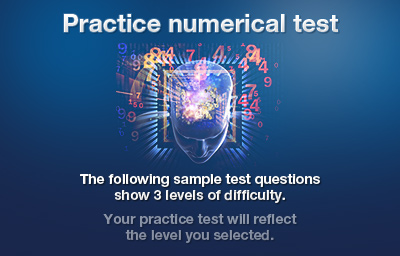•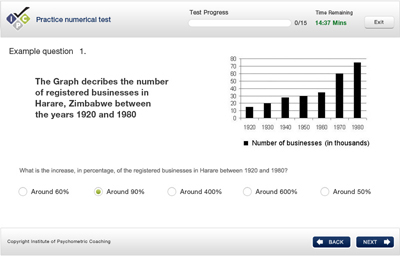•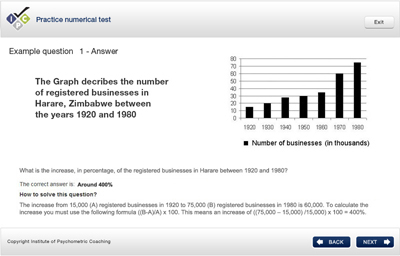•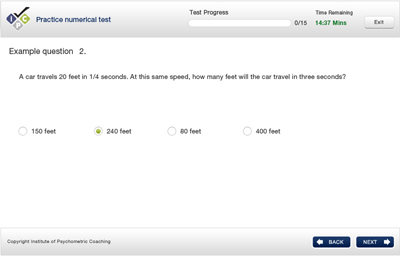•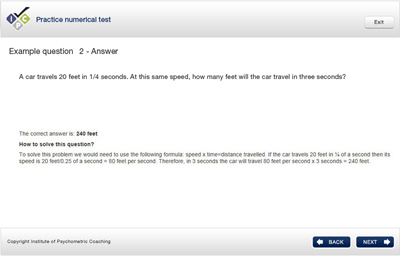•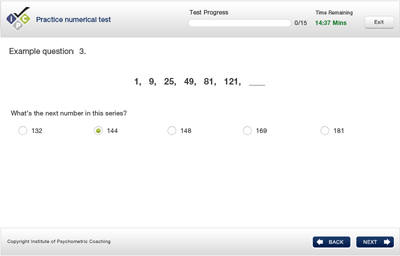•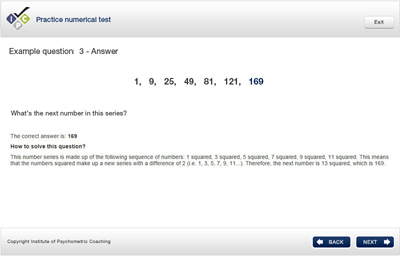•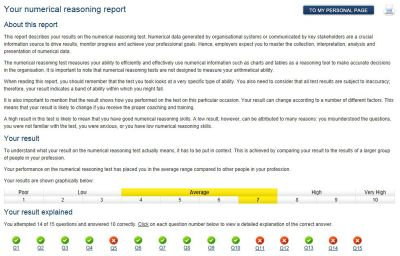## Important numerical aptitude test information:

• The numerical aptitude test is timed and, although various tests may have different time limits, you should expect to be given approximately 50–60 seconds per question.
• The numerical aptitude test is designed so only 1% to 2% of test-takers can correctly answer all questions within the time limit. The most difficult questions are placed at the end of the test.
• Basic numerical aptitude tests measure your arithmetical skills and your ability to do basic calculations, such as percentages.
• Numerical aptitude tests for professional and managerial roles don’t measure your arithmetical skills, but your ability to use numerical information to make business-related decisions. Hence, you are usually allowed to use a simple calculator. The information on whether you are allowed to use a calculator or not will always be given to you prior to taking the test. In most cases, you will not even need to use a calculator to solve the test questions.